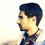# Stuck in Remainders!

Consider the following division, $\frac{x+1}{2x+2}=\frac{\frac{1}{2}(2x+2)}{2x+2}=\frac{1}{2}$ So, dividing $x+1$ by $2x+2$ leaves no remainder. [Of course, $x$ cannot be $-1$ here.]

Now, substituting $x=4$ in the expressions gives us two integers 5 and 10. But, $5=0 \times 10 +5$ We're getting 5 as a remainder now!

Why are these two ways of defining remainders not congruent?Note by Atomsky Jahid
4 years ago

This discussion board is a place to discuss our Daily Challenges and the math and science related to those challenges. Explanations are more than just a solution — they should explain the steps and thinking strategies that you used to obtain the solution. Comments should further the discussion of math and science.

When posting on Brilliant:

• Use the emojis to react to an explanation, whether you're congratulating a job well done , or just really confused .
• Ask specific questions about the challenge or the steps in somebody's explanation. Well-posed questions can add a lot to the discussion, but posting "I don't understand!" doesn't help anyone.
• Try to contribute something new to the discussion, whether it is an extension, generalization or other idea related to the challenge.
• Stay on topic — we're all here to learn more about math and science, not to hear about your favorite get-rich-quick scheme or current world events.

MarkdownAppears as
*italics* or _italics_ italics
**bold** or __bold__ bold
- bulleted- list
• bulleted
• list
1. numbered2. list
1. numbered
2. list
Note: you must add a full line of space before and after lists for them to show up correctly
paragraph 1paragraph 2

paragraph 1

paragraph 2

[example link](https://brilliant.org)example link
> This is a quote
This is a quote
    # I indented these lines
# 4 spaces, and now they show
# up as a code block.

print "hello world"
# I indented these lines
# 4 spaces, and now they show
# up as a code block.

print "hello world"
MathAppears as
Remember to wrap math in $$ ... $$ or $ ... $ to ensure proper formatting.
2 \times 3 $2 \times 3$
2^{34} $2^{34}$
a_{i-1} $a_{i-1}$
\frac{2}{3} $\frac{2}{3}$
\sqrt{2} $\sqrt{2}$
\sum_{i=1}^3 $\sum_{i=1}^3$
\sin \theta $\sin \theta$
\boxed{123} $\boxed{123}$

## Comments

Sort by:

Top Newest

Even though the same word "remainder" is used, you have to bear in mind what the definition in each of these contexts are.

Staff - 4 years ago

Log in to reply

@Brian Charlesworth Would you help me with this?

- 4 years ago

Log in to reply

With proper fractions, (i.e., where the numerator is strictly less than the denominator), the remainder is just the numerator itself, so in your general case,

$x + 1 = 0 \times (2x + 2) + (x + 1)$,

i.e, $x + 1$ divided by $2x + 1$ is $0$ with remainder $x + 1$. Defined this way, a proper fraction does have a remainder, which avoids the contradiction you mention.

- 4 years ago

Log in to reply

I understand your definition. But, is it the proper one? What I've found in the Wikipedia is the following.

"Given two polynomials f(x) (the dividend) and g(x) (the divisor), asserts the existence and the uniqueness of a quotient q(x) and a remainder r(x) such that $f(x)=q(x)g(x)+r(x) ; and, r(x)=0 ,or, deg(r) < deg(g)$"

But, $2x+2$ and $x+1$ both are first-degree polynomials!

Again, I solved some problems where I had to resort to the algebraic manipulation I mentioned in this note.

In an algebra problem, I had to do the following. $R(x)=x^5+\frac{1}{2}x^3+x^2-\frac{1}{2}x+\frac{5}{2}$ $R(x)=(x^2-\frac{1}{2})(x^3+x+1)+3$ $R(x)=x^2(x^3+x+1)-\frac{1}{2}(x^3+x+1)+3$ Then, I had to say $R(x)$ divided by $x^3+x+1$ leaves a remainder of 3.

So, basically we cannot incorporate your definition, can we?

- 4 years ago

Log in to reply

Does the Wikipedia definition assume the $deg(f) \gt deg(g)$? In any event, it also depends on what field we're working with. If we are working with rationals we can indeed say that $x + 1 = \dfrac{1}{2}(2x + 2) + 0$, which satisfies the Wikipedia definition.

In your example where you substituted $x = 4$, this would translate to $5 = \dfrac{1}{2} \times 10 + 0$, but this is not how we normally deal with remainders over the integers, so I think this is the source of the "incongruence" you mention in your question.

(If $deg(f) \lt deg(g)$ then we would necessarily have $q(x) = 0$ and $r(x) = f(x)$, in which case the Wikipedia definition would be satisfied.)

- 4 years ago

Log in to reply

In our case $deg(f) = deg(g)$. And, the definition assumes $deg(f) \geq def(g)$. [This is also the case in Brilliant wiki about polynomial division.]

I also forgot to mention one thing about your first comment. You said, "where the numerator is strictly less than the denominator". But, notice that $x+1$ is strictly greater than $2x+2$ $\forall x < -1$.

- 4 years ago

Log in to reply

Dear sir, can you please help https://brilliant.org/discussions/thread/can-this-limit-be-cancelled/?ref_id=1368476

Thank You!

- 4 years ago

Log in to reply

×

Problem Loading...

Note Loading...

Set Loading...